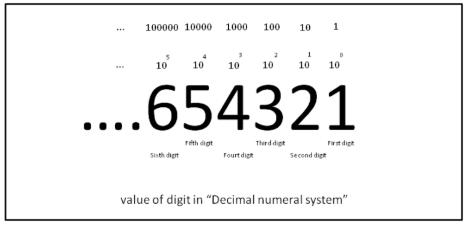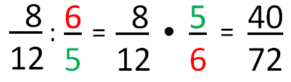Courses

# Decimal Numbers Class 7 Notes | EduRev

## Mathematics (Maths) Class 7

Created by: Praveen Kumar

## Class 7 : Decimal Numbers Class 7 Notes | EduRev

The document Decimal Numbers Class 7 Notes | EduRev is a part of the Class 7 Course Mathematics (Maths) Class 7.
All you need of Class 7 at this link: Class 7

What are Decimal Numbers?
We know the different types of number systems like natural numbers (1,2,3….), whole numbers (0,1,2,3….) and integers (….-2,-1,0,1,2……) used in mathematical expressions. Integers also consist of fractions like 3/5, 9/2 etc. Well, the decimal numbers are actually a type of fractions.

As the name implies (Greek: Deca = 10) decimal numbers are fractions with the divisor as 10 or multiples of 10. A unit is represented as 1. If I ask you to divide 1 into 10 equal parts then each part is called one-tenth of the original,

• One tenth is 1 unit divided into 10 equal parts = 1/10
• Similarly, One hundredth is 1 unit divided into 100 equal parts = 1/100
• One thousandth is 1 unit divided into 1000 equal parts = 1/1000

and so on as the value of the divisor increases, the output gets smaller and smaller.

Decimal PointThe decimal point is the most important concept here. This point is used to denote the number has been divided by 10 or its multiples. For example,
1/10 = .1  (read as point one)
1/100 = .01 (read as point zero one)
1/1000 = .001 (read as point zero zero one)
thus 377/1000 = .377 (read as point three seven seven) and  12966/100=129.66 (read as one hundred and twenty-nine point six six). The number placed to the left of the decimal point is larger than one and the number placed to the right is less than one.

The decimal point is placed to the right of the unit’s place in the number. This point makes it easy to read and represent a number or fraction. For example, 978/1000 can be easily represented as 0.978 and instead of reading nine hundred and seventy-eight divided by one thousand we can read it as point nine seven eight without changing its meaning.

Representation
To represent a decimal fraction with a decimal point consider the unit place of the dividend or the numerator. Count the number of zeroes in the denominator and then moving left from the unit place of the numerator place the point after an equal number of digits.
For example, consider the fraction  2489/100. Here the number of zeroes in the denominator is 2. So moving left from the unit place of the numerator we place the point after 2 digits i.e. left of 9 and 8. So, 2489/100=24.89 (read as twenty-four point eight nine).
Consider the fraction 3/1000. Here the denominator has 3 zeroes. But in the numerator, we have only the unit place. In such a case from the unit place, we move 3 places left using zeroes ahead of the numerator. So, taking the unit place and moving left we cover 1 point, then we add one zero and cover 2 points, again we add one more zero and thus cover 3 points.
So, 3/1000=.003 (read as point zero zero three). Let’s take a look at some of the mathematical operations using decimal numbers.

Addition of decimal numbers follows the same rules as the addition of integers. Make sure to put the decimal point at the required location. For example, 273.05+ 21.705= 294.755

Subtraction
Rules of subtracting decimal numbers are the same as that of subtracting integers. Make sure to put the decimal point at the correct location. For example, 273.05-21.30=251.75

The topics of multiplication and division of decimal numbers have been dealt with in another discussion.

Solved Question
Ques. The sum of two numbers is 31.021. If one of them is 11.56,  then the other number is
1. 19.461
2. 17.461
3. 18.461
4. 19.561
Sol: 31.021 – 11.56 = 19.461

Division of Fractions
Division of fractions is a simple operation. You will be surprised to know that division of one fraction by other fraction is actually a multiplication. Multiply the first fraction with the reciprocal of the other fraction to obtain the final result.

Reciprocal of a FractionThe reciprocal of a number “a” is given as “1/a”. Reciprocal of a fraction basically means changing the places of the numerator and the denominator. Consider the fraction 3/4. Reciprocal of this fraction is obtained by changing the places of 3 and 4. So the reciprocal of 3/4 is 4/3. Similarly, reciprocal of 7/9 and 1/6 is 9/7 and 6/1 respectively.

Note that changing the places of the numerator and denominator changes the value of the fraction entirely. Simplification of the operation of division is the only aim here for which we do this. So, the steps for the division of fractions include, firstly change the second fraction to its reciprocal and then using the rules of multiplication obtain the final result.
For example,

• 3/4 divided by 1/3. To solve this, we first obtain the reciprocal of 1/3. Interchanging the numerator and denominator we get 3/1. Now, multiplying the fractions we get 3/4 × 3/1 = 9/4.
• 3/4 divided by 1/2. Now again we take the reciprocal of the second fraction we get 2/1. Using rules of multiplication we get, 3/4 × 2/1. Canceling the common terms here we get 3/2.

Division of Whole Numbers by Fractions and Vice Versa
The use of fractions to divide whole numbers or vice versa is done to obtain ratios.

• For example, if asked to divide 6 by 3/4, we convert 6  to a fraction as studied to 6/1. We take reciprocal of 3/4 i.e. 4/3. Using the rules of multiplication we get 6/1 × 4/3. Canceling the common term we get 2/4 which is equal to 1/2.
• Again consider an example of dividing 7/8 by 4. To do so, we first convert the whole number into a fraction i.e. 4/1. We take reciprocal of the second fraction, so we get 1/4. Using the rules of multiplication of fractions we get 7/8 ×1/4=7/32

So to divide a whole number by a fraction or vice versa we convert the whole number to a fraction, take the reciprocal of the second fraction, multiply both the fractions using rules of multiplication and obtain the results.

Division of Mixed Fractions
Mixed fractions represented as xy/z can be converted to fractions using the formula (z.x+y)/z and then division can be carried out on these fractions as studied. Consider the example, divide 23/5  by 31/4.
We convert the mixed fractions into fractions. We get 2 fractions 13/5 and 13/4. Now we take reciprocal of the second fraction and multiply 13/5 × 4/13. Canceling common terms and we get 4/5.

Solved Question
Ques: Divide 7 by 23/4
Sol: Now converting the whole number to a fraction and converting mixed fraction to regular fraction
= 7/1 and 11/4
taking reciprocal of the second fraction and multiplying,
7/1 × 4/11
= 28/11
= 2 6/11

Offer running on EduRev: Apply code STAYHOME200 to get INR 200 off on our premium plan EduRev Infinity!

,

,

,

,

,

,

,

,

,

,

,

,

,

,

,

,

,

,

,

,

,

;